#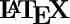Support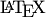has been integrated into this site, making it easy to include mathematics into the pages you edit. This is not a standard feature of PmWiki, so it is not covered in the usual documentation on text formatting rules. This page covers the inclusion ofcode and addresses some of the complications that arise.

The syntax used to produceoutput is basically what you would expect. For example, the formula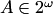is produced by the code $A\in 2^\omega$. The usual markup is also provided for display mathematics. In addition, text delimited by \@ and \@ is compiled asin text mode and included inline. Text beween \@@ and \@@ is compiled in text mode too, but then centered in the same way as display mathematics.

The following table summerizes the different ways to includein pages on this site.Markup
 inline mathematics $...$ or $$...$$ display mathematics $$...$$ or $...$ inline text \@...\@ display text \@@...\@@

## Important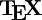nical Notes

1.code is compiled in documentclass article with amssymb and amsmath loaded. Please request macros and additional packages which you would find particularly useful.

2. I have tried to ensure that the vertical alignment of inline formulas is correct on browsers that support CSS (cascading style sheets). Please contact me if the formulas below do not align well on your browser.

Formulas like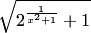and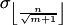should be aligned with the surrounding text.

3. The first matching end delimiter is always interpreted as the end of theexpression. Thus, $\$$ produces an error but $$\$$ produces. 4. To produce a dollar sign type [=$=]. This prevents the Wiki engine from interpreting it as part of an inline formula.

5. You must explicitly change the font of inlineto match changes in the surrounding text font. For example, if you enter [+++'''Let $A\in 2^\omega$ be 1-random.'''+++] then you get

Letbe 1-random.

In order to generate

Let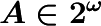be 1-random.

you should enter [+++'''Let \@\boldmath \LARGE $A\in 2^\omega$\@ be 1-random.'''+++]. Note that math font information is generally set before entering math mode, so the \@...\@ markup is usefull here.

Although not perfect, there is a fairly reasonable correspondence between font sizes inand in the text markup on this site. The following tables suggests the code to use in various situations.

 Matching Font Sizes
 PmWiki markup Corresponding[+++...+++] \LARGE [++...++] \Large [+...+] \large [-...-] \footnotesize [--...--] \scriptsizein Headings
 PmWiki markup Corresponding! \boldmath\LARGE or \bf\LARGE !! \boldmath\Large or \bf\Large !!! \boldmath\large or \bf\large